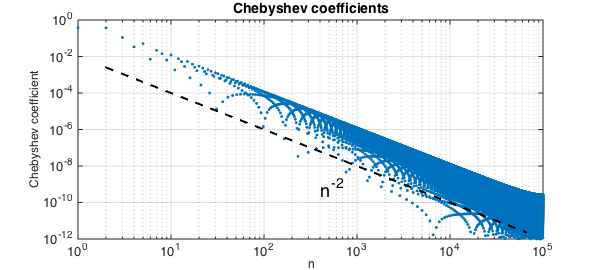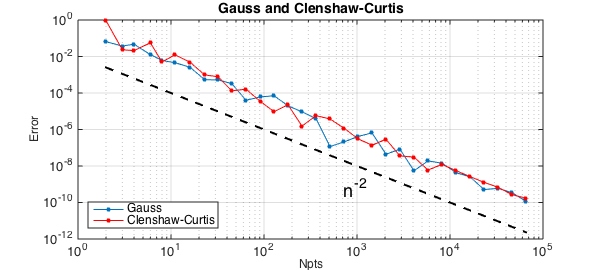As pointed out first by Folkmar Bornemann, the Gauss and Clenshaw-Curtis quadrature formulas converge at a rate one power of $n$ faster than one might expect. For example, consider the function $f(x) = |x-.3|$. Its Chebyshev coefficients decrease at the rate $n^{-2}$:

clf
x = chebfun('x');
f = abs(x-.3);
fc = chebfun(@(x) f(x),1e5);
LW = 'linewidth'; FS = 'fontsize'; MS = 'markersize';
plotcoeffs(fc,'loglog','.',MS,8), axis([1 1e5 1e-12 1])
xlabel('n',FS,12), ylabel('Chebyshev coefficient',FS,12)
nn = round(2.^(1:.5:16));
hold on, loglog(nn,.01*nn.^(-2),'--k',LW,2)
text(4e2,.5e-9,'n^{-2}',FS,18)Since the integral of an $O(n^{-2})$ tail is normally of size $O(n^{-1})$, you might expect these quadrature formulas to have accuracy $O(n^{-1})$. But in fact, it is $O(n^{-2})$ again:

clf, exact = sum(f);
errg = []; errc = [];
nn = round(2.^(1:.5:16));
for n = nn
[s,w] = legpts(n);
Igauss = w*f(s);
errg = [errg abs(Igauss-exact)];
[s,w] = chebpts(n);
Iclenshawcurtis = w*f(s);
errc = [errc abs(Iclenshawcurtis-exact)];
end
loglog(nn,errg,'.-',LW,1,MS,16), grid on
axis([1 1e5 1e-12 1]), hold on
xlabel('Npts',FS,12), ylabel('Error',FS,12)
loglog(nn,errc,'.-r',LW,1,MS,16)
title('Gauss and Clenshaw-Curtis',FS,14)
legend('Gauss','Clenshaw-Curtis','location','southwest')
loglog(nn,.01*nn.^(-2),'--k',LW,2)
text(7e2,.5e-9,'n^{-2}',FS,18)Xiang and Bornemann develop theorems that establish that this effect occurs generally . The reason is not hard to explain once you know to look for it. Both the Clenshaw-Curtis and Gauss formulas will integrate $T_m(x)$ incorrectly for $m\gg n$: instead of giving you the integral of $T_m$, they'll give you the integral of some lower-degree polynomial alias of $T_m$. But $T_m$ is highly oscillatory, with integral $O(n^{-1})$, and most of the time those aliases are highly oscillatory too, also with integral $O(n^{-1})$. So the error committed in integrating $T_m$ is typically of size $O(n^{-1})$, not $O(1)$. It's only as big as $O(1)$ for the unlucky values of $m$ that get aliased to a polynomial with a lot of energy at wave number $O(1)$, and only a fraction $O(n^{-1})$ of the values of $m$ have this unlucky property.

Or as Xiang and Bornemann put it: $E_n(T_m)$ is, up to some remainder, periodic in $m$ with a period of $O(n)$ and an average modulus of $O(n^{-1})$.

Xiang and Bornemann point out that for a function with so little smoothness as $|x-.3|$, these convergence results were noted earlier by Riess and Johnson (1971/72) for Clenshaw-Curtis and Davis and Rabinowitz (1984) for Gauss. The general theorems seem to be new, however, and their proofs require careful attention to details.

1. S. Xiang and F. Bornemann, On the convergence rates of Gauss and Clenshaw-Curtis quadrature for functions of limited regularity, SIAM Journal on Numerical Analysis, 50 (2012), 2581-2587.